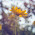## Sunday, December 30, 2012

### USACO - Superprime Rib

As you know reading the problem statement carefully is crucial to find the first hints for solving the problem. After I noticed the structure of the problem, I found something important. Each number you print in the output, what is the first digit of that? 2, 3, 5 or 7, isn't it? Another thing is that you can find super prime numbers with length n, from the super prime numbers of length (n-1). If we have super prime numbers of length (n-1) which digits can we add to construct super prime numbers with length n? We can add 1, 3, 7 or 9 to the end of a super prime number of length (n-1) to build a number of length n which could be a super prime number of length n(if we add other digits to the end of that number it would be divisible by 2 or 5). After doing this we check whether it is a prime or not? To check whether a number is prime or not I refer you to my previous post about usaco-prime palindromes.

#### 1 comment:

1.i think recursive make faster and clear answer.